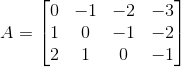Construct a 3×4 matrix A = [ai j] whose elements ai j are given by ai j = i – j

Asked by Aaryan | 1 year ago |  46

##### Solution :-

Given ai j = i – j

Let A = [ai j]2×3

So, the elements in a 3×4 matrix are

a11, a12, a13, a14, a21, a22, a23, a24, a31, a32, a33, a34

a11 = 1 – 1 = 0

a12 = 1 – 2 = – 1

a13 = 1 – 3 = – 2

a14 = 1 – 4 = – 3

a21 = 2 – 1 = 1

a22 = 2 – 2 = 0

a23 = 2 – 3 = – 1

a24 = 2 – 4 = – 2

a31 = 3 – 1 = 2

a32 = 3 – 2 = 1

a33 = 3 – 3 = 0

a34 = 3 – 4 = – 1

Substituting these values in matrix A we get,Answered by Aaryan | 1 year ago

### Related Questions

#### Find the values of a, b, c and d from the equations

Find the values of a, b, c and d from the equations:

#### Find x, y, a and b if

Find x, y, a and b if

#### Find x, y, a and b if

Find x, y, a and b if

Construct a 4 × 3 matrix A = [ai j] whose elements ai j are given by $$a_{i j} = \dfrac{(i – j)}{(i + j)}$$